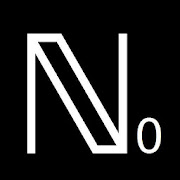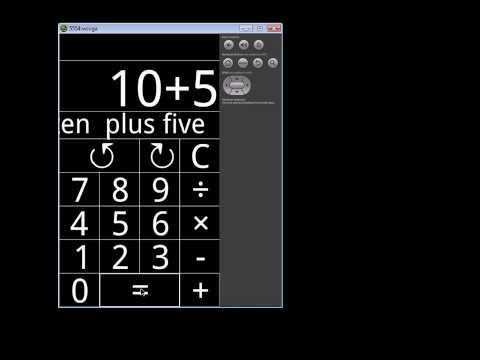# Whole CalculatorEveryone
22How to write Big Numbers in English:
Small calculator, within the set of Natural Numbers.
Designed to the time of formation of the students in which they can add and multiply, and subtract or divide with integers and positive results.
When the results come out of this range, the calculator warns of this: The negative results of the subtractions, and remainder results in the division.
The calculator warns of the need for a better tool for these calculations.
And new knowledge.
At the same time write the spelling of numbers and operations.

Calculator in the set of the natural numbers (ℕ).
And is able to write all the natural numbers with words.
When the result leaves the set of natural numbers, this calculator points to the need of to upgrade to a calculator with more features. ( ℤ calculator or ℚ calculator).
Type the numerical operations and results in words.

Writing numbers :
The app writes correctly from from the one (10^0) until the centillion (10^303).
Numbers converted in words in a text scroller: Marquee sliding text.

Video: How looks a Centillion?:

Also useful to explore cyclic numbers:
http://en.wikipedia.org/wiki/Cyclic_number

142857 * 5 = 714285; 142857 * 6 = 857142; ......

More cyclic numbers:
(10^6 - 1) / 7 = 142857 (6 digits)
(10^16 - 1) / 17 = 0588235294117647 (16 digits)
(10^18 - 1) / 19 = 052631578947368421 (18 digits)
(10^22 - 1) / 23 = 0434782608695652173913 (22 digits)

.....and more

Post of the first publication of this: "Whole Calculator" at Nummolt Blog
http://nummolt.blogspot.com/2014/03/whole-calculator-android-app-this-week.html

Double Struck Capital - nummolt apps:
http://www.nummolt.com

Type in the keyboard, and the spelling of the numbers or operations appears in the upper screen converted in words.

Meaning of the buttons:
↺ "Undo" (undo the last action)
↻ "Redo" (redo the last undone action)
C "Clear all" (clears all screens and empty the inner list of actions)
÷ "Divide" (adds the character ÷ on the screen and add this action in the inner list of actions)
× "Multiply" (adds the character × on the screen and add this action in the inner list of actions)
- "Minus" (adds the character - on the screen and add this action in the inner list of actions)
+ "Plus" (adds the character + on the screen and add this action in the inner list of actions)
(The same with all the numerical buttons)
= "Equal" (adds the character = on the screen, add this action in the inner list of actions and performs the operation)

Based in part on the "Really Big numbers" mathcats application, made in collaboration between Wendy Petti ( www.mathcats.com ) and Maurici Carbó ( www.nummolt.com ) in 2003
http://www.mathcats.com/explore/reallybignumbers.html

Writing numbers:
The program uses the: SHORT SCALE: (British and US) Map of usages:
http://en.wikipedia.org/wiki/File:World_map_of_long_and_short_scales.svg
(Valid and useful in the blue colored countries of this map)

Thousands separator conforms to: ISO/IEC 80000-1:2009

Double-struck capital N is: ℕ
In utf8: (U+2115): ℕ
As Java (+ Android) source char: "\u2115": ℕ
In mathematical texts ℕ Represents the set of the natural numbers. (May or may not include zero).

**** from Merlot: http://www.merlot.org/merlot/viewMaterial.htm?id=850283

Registered at the MathTools database:
http://mathforum.org/mathtools/tool/235179/
Courses:
Kindergarten: Addition, Subtraction, Whole numbers, Counting, Writing numbers, Place value
Math 1: Addition, Subtraction, Whole numbers, Counting, Writing numbers, Place value
Math 2: Addition, Subtraction, Multiplication, Division, Whole numbers, Counting, Writing numbers, Place value
Math 3: Addition, Subtraction, Multiplication, Division, Whole numbers, Place value
Math 4: Addition, Subtraction, Multiplication, Division, Whole numbers
Collapse

Review Policy
3.3
22 total
5
4
3
2
1

## What's New

vn: 1.0.7 vc: 8 sdk29 Update Android 10
Collapse

Updated
October 11, 2020
Size
636k
Installs
1,000+
Current Version
1.0.7
Requires Android
4.0 and up
Content Rating
Everyone
Permissions
Offered By
Double Struck Capital
Developer
Maurici Carbó C/ Sant Antoni Maria Claret, 324 Barcelona 08041 S.PAINSee more